• 通常我们求解一维卷积或者二维卷积都是采用模板平移的方法，今天我们介绍一种新的求解方法，可以一次性求出所有的结果。一维卷积卷积定义对于两个长度分别为m和n的序列x(i)和g(i)有， ...循环矩阵参考百度百科：
通常我们求解一维卷积或者二维卷积都是采用模板平移的方法，今天我们介绍一种新的求解方法，可以一次性求出所有的结果。

一维卷积

卷积定义

对于两个长度分别为m和n的序列x(i)和g(i)有， h(i)=x(i)∗g(i)=∑jx(j)g(i−j)<!--//--><![CDATA[//><!--
h(i)=x(i)*g(i)=\sum_{j}x(j)g(i-j)
//--><!]]>
上式给出了长度为N=m+n-1的输出序列。称为一维情况下的卷积公式。

循环矩阵

参考百度百科： 在线性代数中，循环矩阵是一种特殊形式的 Toeplitz矩阵，它的行向量的每个元素都是前一个行向量各元素依次右移一个位置得到的结果。由于可以用离散傅立叶变换快速解循环矩阵，所以在数值分析中有重要的应用。

定义

形式为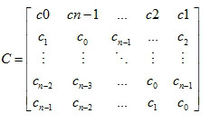的 矩阵 C 就是循环矩阵。

性质

循环矩阵的性质：
1. 循环矩阵遵循代数运算法则。对于两个循环矩阵 A 与 B 来说，A + B 也是循环矩阵。AB 也是循环矩阵，并且 AB=BA。
2. 循环矩阵的特征向量矩阵是同样维数的离散傅立叶变换矩阵，因此循环矩阵的特征值可以很容易地通过快速傅立叶变换计算出来。

循环矩阵求解卷积

按照定义，卷积后的序列长为N=m+n-1;
于是分别用0扩充序列x,g，分别为： xp(i)={x(i)01⩽x⩽mm<i⩽N<!--//--><![CDATA[//><!--
x_p(i)=\left\{\begin{matrix}
x(i) & 1\leqslant x \leqslant m\\
0& m<i\leqslant N
\end{matrix}\right.
//--><!]]>gp(i)={g(i)01⩽x⩽nn<i⩽N<!--//--><![CDATA[//><!--
g_p(i)=\left\{\begin{matrix}
g(i) & 1\leqslant x \leqslant n\\
0&n<i\leqslant N
\end{matrix}\right.
//--><!]]>
由于是用g去卷积f,所以构造g的循环矩阵： G=⎡⎣⎢⎢⎢⎢⎢⎢⎢gp(1)gp(2)⋮gp(N)gp(N)gp(1)⋮gp(N−1)gp(N−1)gp(2)⋮gp(N−2)⋯⋯⋱⋱gp(2)gp(3)⋮gp(1)⎤⎦⎥⎥⎥⎥⎥⎥⎥<!--//--><![CDATA[//><!--
G=\begin{bmatrix}
g_p(1) &g_p(N) &g_p(N-1)  &\cdots   & g_p(2) \\
g_p(2)  &g_p(1) &g_p(2)  &\cdots   &g_p(3)  \\
\vdots  & \vdots  & \vdots  &\ddots   &\vdots  \\
g_p(N) &g_p(N-1)&g_p(N-2)  &\ddots  & g_p(1)
\end{bmatrix}
//--><!]]>
则h=G⋅xp=⎡⎣⎢⎢⎢⎢⎢⎢⎢gp(1)gp(2)⋮gp(N)gp(N)gp(1)⋮gp(N−1)gp(N−1)gp(2)⋮gp(N−2)⋯⋯⋱⋱gp(2)gp(3)⋮gp(1)⎤⎦⎥⎥⎥⎥⎥⎥⎥⎡⎣⎢⎢⎢⎢⎢xp(1)xp(2)⋮xp(N)⎤⎦⎥⎥⎥⎥⎥<!--//--><![CDATA[//><!--
h=G\cdot x_p=\begin{bmatrix}
g_p(1) &g_p(N) &g_p(N-1)  &\cdots   & g_p(2) \\
g_p(2)  &g_p(1) &g_p(2)  &\cdots   &g_p(3)  \\
\vdots  & \vdots  & \vdots  &\ddots   &\vdots  \\
g_p(N) &g_p(N-1)&g_p(N-2)  &\ddots  & g_p(1)
\end{bmatrix}\begin{bmatrix}
x_p(1)\\
x_p(2)\\
\vdots \\ x_p(N)
\end{bmatrix}
//--><!]]>
则h就是卷积后的向量。

二维卷积

卷积定义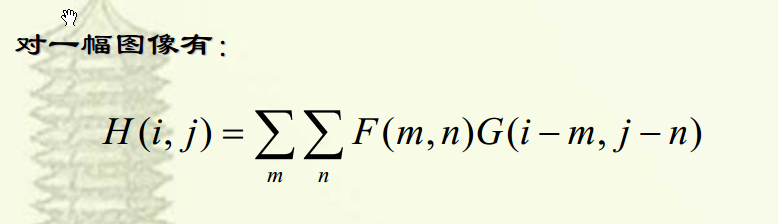循环矩阵求解方法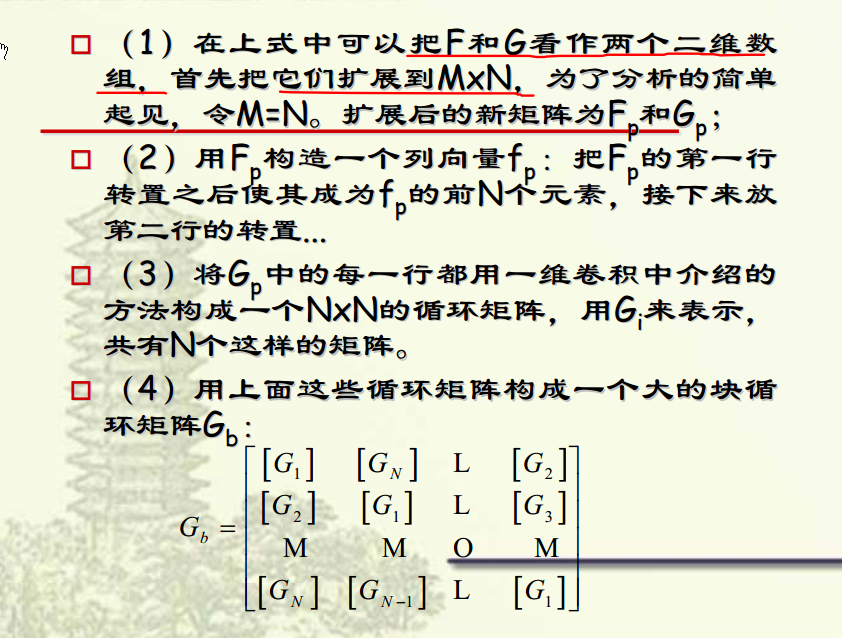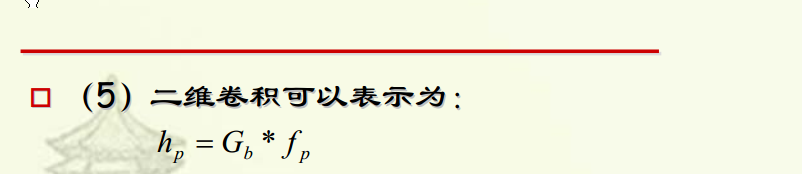举例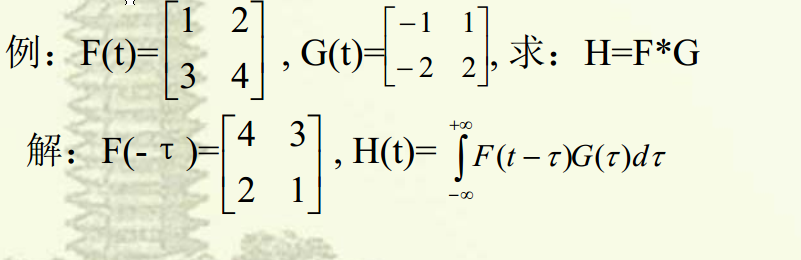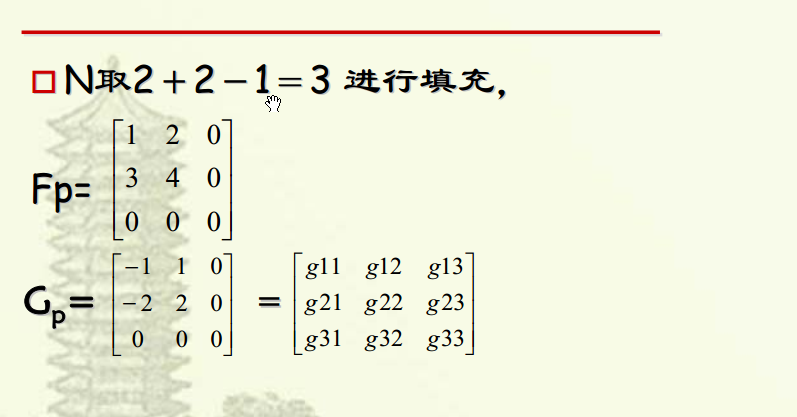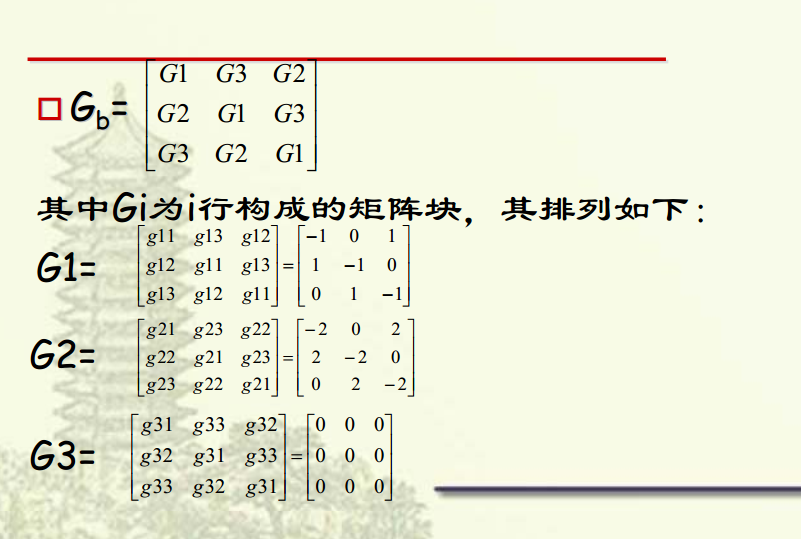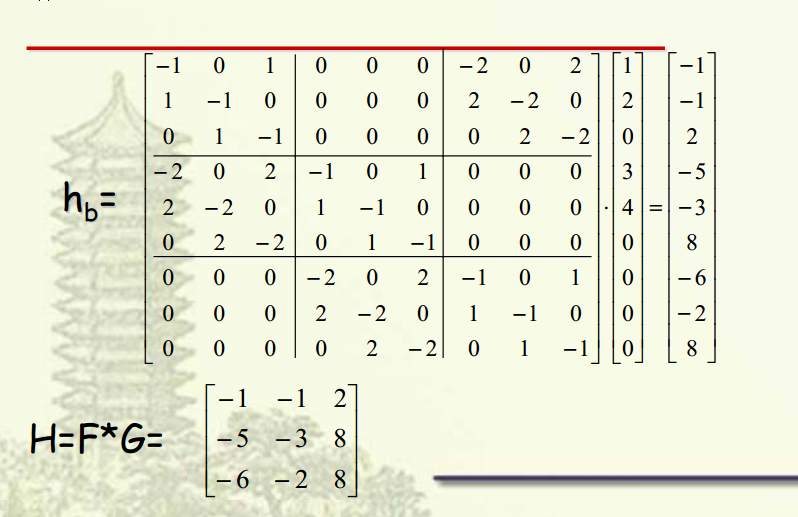参考文献

北大高级遥感数字图像处理（硕士生课程）
离散卷积与自相关展开全文图像处理
• 嵌套循环求解矩阵运算，在主函数中定义一个二维数组，将数据存放到二维数组中，判断数组中的元素是否符合条件，将符合条件的数据添加到总和中，输出总和。
• 对于许多递推题目，由于数据范围太大直接循环写会爆掉，这时首先想到的就是矩阵优化，把递推式转换成01矩阵通过快速幂优化。  比如最简单的斐波纳挈，如果n非常大，循环递推肯定是不合适的，那么可以考虑下面的...
 对于许多递推题目，由于数据范围太大直接循环写会爆掉，这时首先想到的就是矩阵优化，把递推式转换成01矩阵通过快速幂优化。
比如最简单的斐波纳挈，如果n非常大，循环递推肯定是不合适的，那么可以考虑下面的公式  (f[n],f[n-1])=(f[n-1],f[n-2])*A; 这里的A是一个01矩阵，此时的A={1,1,1,0} 2*2的矩阵，    可想而知 f = A的n-2次幂*(f,f);   形如斐波纳挈的递推公式转换矩阵都很简单， 顺便附上POJ3070的 斐波纳挈转矩阵快速幂的代码

#include<cstdio>
#include<cstring>
#include<iostream>
#define MOD 10000
using namespace std;

struct mac
{
int frob;
}pri,unit;

mac power(mac x,mac y)
{
mac temp;
for(int i=0;i<2;i++)
{
for(int j=0;j<2;j++)
{
temp.frob[i][j]=0;
for(int k=0;k<2;k++)
{
temp.frob[i][j]=(temp.frob[i][j]+x.frob[i][k]*y.frob[k][j])%MOD;
}
}
}
return temp;
}
void eachother(int n)
{
pri.frob=pri.frob=pri.frob=1;
pri.frob=unit.frob=unit.frob=0;
unit.frob=unit.frob=1;
while(n)
{
if(n&1)
{
unit = power(unit,pri);
}
pri = power(pri,pri);
n >>= 1;
}
printf("%d\n",unit.frob);
}
int main()
{
int n;
while(scanf("%d",&n)!=EOF)
{
if(n==-1) break;
if(n==0)
{
printf("0\n");
continue;
}
if(n==1||n==2)
{
printf("1\n");
continue;
}
eachother(n);
}
return 0;
}


展开全文• 主要思路是找到行和列的规律，再用for循环的嵌套求解。 #include"stdio.h" int main(void) { int n; scanf("%d",&n); static int a=1; static int b=1; for(int row=1;row<n+1;row++) { a+=row-1...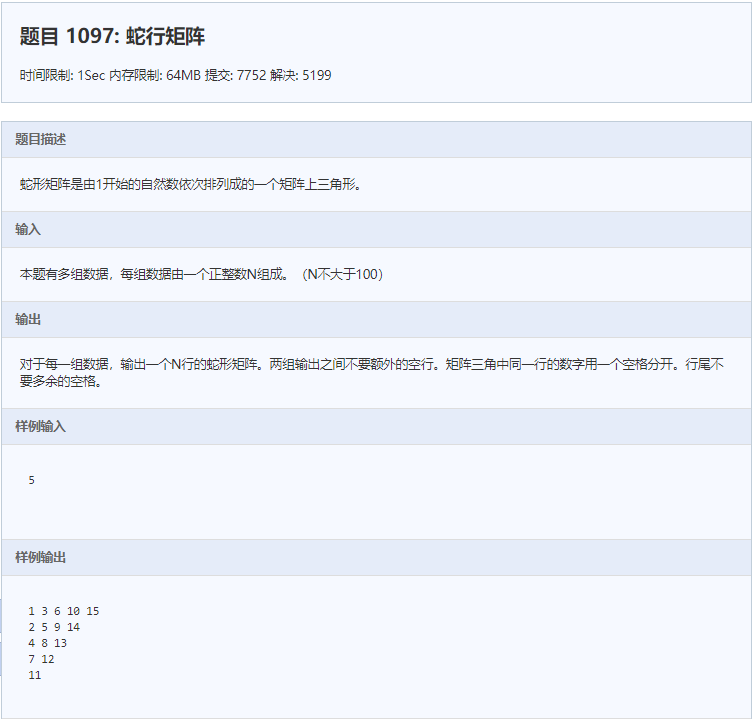主要思路是找到行和列的规律，再用for循环的嵌套求解。

#include"stdio.h"
int main(void)
{
int n;
scanf("%d",&n);
static int a=1;
static int b=1;
for(int row=1;row<n+1;row++)
{
a+=row-1;
printf("%d",a);
b=a;
for(int i=2;i<n-row+2;i++)
{
putchar(' ');
printf("%d",(b+row+i-1));
b+=row+i-1;

if(i==n-row+1)
printf("\n");
}
}
return 0;
} 


展开全文c语言 算法
• 这段时间做了些工作，在Mac下基于opencv做了单应性矩阵求解，下面的文档中给出了循环读取文件的代码，读出文件以后两两做siftImageAlignment，求出了几何校正后影像result和单应性矩阵H。import numpy import os ...
这段时间做了些工作，在Mac下基于opencv做了单应性矩阵的求解，下面的文档中给出了循环读取文件的代码，读出文件以后两两做siftImageAlignment，求出了几何校正后影像result和单应性矩阵H。

import numpy
import os
import math
import cv2
def image_file(file_path):
if os.path.exists(file_path):
print("files exists")
else:
print("no files exists")

image_dir = []
files = os.listdir(file_path)
for fi in files:
fi_d = os.path.join(file_path, fi)
if os.path.isdir(fi_d):
print(os.path.join(file_path, fi_d))
image_file(fi_d)
else:
if fi.endswith('JPG'):
print(os.path.join(file_path, fi_d))
image_dir.append(fi_d)
else:
print("error file")
print len(image_dir)
return image_dir

# save_path change
def save_file(img_dir,save_image,save_path):
[dirname,filename] = os.path.split(img_dir)
save_image_ = os.path.join(save_path,filename)
cv2.imwrite(save_image_, save_image)
return save_image_

# extract sift_feature and keypoints
def sift_kp(image):
print 'sift_kp'
gray_image = cv2.cvtColor(image,cv2.COLOR_BGR2GRAY)
sift = cv2.xfeatures2d_SIFT.create()
kp,des = sift.detectAndCompute(image,None)
kp_image = cv2.drawKeypoints(gray_image,kp,None)
return kp_image,kp,des

# good match
def get_good_match(des1,des2):
print 'get_good_match'
bf = cv2.BFMatcher()
matches = bf.knnMatch(des1, des2, k=2)
good = []
for m, n in matches:
if m.distance < 0.75 * n.distance:
good.append(m)
return good

- # image alignment
def siftImageAlignment(img1,img2):
print 'siftImageAlignment'
_,kp1,des1 = sift_kp(img1)
_,kp2,des2 = sift_kp(img2)

goodMatch = get_good_match(des1,des2)
print 'get_good_match_end'

   if len(goodMatch) > 4:
ptsA= numpy.float32([kp1[m.queryIdx].pt for m in goodMatch]).reshape(-1, 1, 2)
ptsB = numpy.float32([kp2[m.trainIdx].pt for m in goodMatch]).reshape(-1, 1, 2)
ransacReprojThreshold = 4
H, status =cv2.findHomography(ptsA,ptsB,cv2.RANSAC,ransacReprojThreshold);
print(H)
print 'findHomography'
imgOut = cv2.warpPerspective(img2, HT, (img1.shape,img1.shape),flags=cv2.INTER_LINEAR + cv2.WARP_INVERSE_MAP)
print 'warpPerspective'
return imgOut,H,status

print 'begin'
numbers = 0
K = numpy.array([[3.95, 0, 2.4], [0, 3.95, 1.8], [0, 0, 1]])
result = cv2.imread(image_dir)

 - #circle read image_dir to deal with jpg images
for numbers in range(len(image_dir)-1):
print "this is the circle %d of image_dir" %(numbers)
if len(image_dir) < 1:
print "not enough images"
else :
img1 = result;
result, H, _ = siftImageAlignment(img1, img2)
save_file(image_dir[numbers+1],result,save_path)
print 'result'
print("final end")
展开全文opencv 几何校正
• 本程序因时间问题一直没有修改其通用性，这是一个最大的问题，希望有兴趣的...所以请朋友们在运行的时候，如想修改起先路径请自已的定义的时候自行修改，同时也要修改程序本身的一些相关数据，如循环长度等。。。。。
• 输入一个矩阵的行列数量，生成一个螺旋矩阵，比如输入3， ...依次 从左往右，从上往下，从右往左，从下往上 依次循环。直到填满为止。 其实是有规律的，假如是 N 螺旋，第一圈（最外圈）依次是 从左往右
• 解题思路： 很容易想到每一轮的dp方程： a[i]=(1−1m)a[i]+1ma[i−1=0?n:i−1]a[i]=(1-\frac{1}{m})a[i]+\frac{...注意到转移矩阵是一个循环矩阵，即每一行都是上一行平移一格得到(列也是如此)，而循环矩阵的性质有：
•  * 函数功能: Jacobi行循环法求取n阶实对称矩阵A的所有特征值和特征向量V(:,i)   * 输入参数:   A: n阶实对称矩阵, 算法结束后其第j个对角线元素为A的第j个特征值  n: A的阶数  V: n阶单位矩阵, ...算法 delete 存储 测试
• 域Zp上的循环线性系统的求解方法，张飞，，为了求解域Zp上的循环线性系统,就要求它们系数矩阵的逆阵及Moore-Penrose逆,本文给出域Zp上的循环循环线性系统的一种算法.当循环矩阵非�
• python3 中如何print循环输出标准矩阵前言做拼多多提前批笔试题时，出现不会输出标准矩阵，下去求解后记录一下。一、解决如下代码如下(示例)：# 输出调整为矩形，可以按下面的方式import sysn = int(input())nums=[...
• 前2个方法，都比较简单，刷题时候用过了，但是这次刷题，属于Fibonacci加强版，对时间性能很有要求，使用1或者2方法一直ac不了，所以看了矩阵求解方法。这个方法看了一个下午才明白，所以一定要记录的。3.1、先看...
• 本篇为稀疏矩阵求解算法经典论著<Direct Methods for Sparse Linear System>的<读书笔记 4>Chapter 2 Basic algorithms上一篇主要介绍了稀疏矩阵的数据结构，那么在接下来本章主要包括了其几个基础的...数据挖掘
• 雅可比方法用于求解实对称矩阵的特征值和特征向量,对于实对称矩阵AAA,必有正交矩阵UUU,使得UTAU=DU^{T}AU=DUTAU=D.DDD是一个对角阵,主对角线的元素是矩阵AAA的特征值,正交矩阵UUU的每一列对应于属于矩阵DDD的主对角...算法 c语言
• 思路：循环输出，注意边界控制 ...public class ch2_8_1求解n阶螺旋矩阵问题 { public static void main(String[] args) { // TODO Auto-generated method stub Scanner in=new Scanner(System.in); int...
• python3 中如何如何print循环输出标准矩阵 前言 做拼多多提前批笔试题时，出现不会输出标准矩阵，下去求解后记录一下。 一、解决如下 代码如下（示例）： # 输出调整为矩形，可以按下面的方式 import sys n = int...
• 得出了一个简单的稀疏表示公式： (1)那么自然我们就会得出一个问题，现在我们已知信号y，以及一个过完备字典D，如何才能求解出这个信号的稀疏表示α呢？本文要介绍的匹配追踪算法（Matching Pursuit， MP）就是最...
• 题意：给定x 求解 思路： 由斐波那契数列的两种表示方法， 之后可以转化为 线性表示 F[n] = F[n-1] + F[n-2] ; 同时可以看出 和 是 一元二次方程的两根， a = 1, b = -1 又是之后递推式的系数； 同理这里需要...
• 如果在一个矩阵中，出现了一个数，它在矩阵中的所在的行里是最小的，但在所在的列里是最大的，测称这个数是这个矩阵的一个鞍点。 2、鞍点求解思路 首先先求出第一行中最小的数，然后求该数在所在列是否是...
• 以非循环可达关系为基础, 定义矩阵的计算规则, 使用系统的邻接矩阵来计算可达矩阵。同时首次提出了循环可达关系的分类、二可达关系等, 并设计了求循环可达关系的算法, 且以实例证明了算法的有效性和正确性。在不确定...
• 思路：如果直接利用n做三次矩阵快速幂求解是不对的。因为三次快速幂对1000000007取模，会超精度。所以必须本地处理寻找每层的循环节，最外层最1000000007取模，则找到最外层的循环节是222222224，次外层对222222224...
• 碰到递推果断矩阵快速幂，如果是乘法，就用矩阵快速幂求解指数 记住mod p的时候，指数要用费马小定理 mod p-1 还有如果a%p==0的时候，如果指数为0，需要把指数加上p-1 http://acm.hdu.edu.cn/showproblem.ph
• 存储为矩阵，下面的代码循环总是无法结束，求解，谢谢！ ` vector<vector<char>> grid; string s; string str = ""; while (cin>>s) { vector<char>temp; for (int i = 0; i (); i++) { if ...c++
• 将gg(n)视为一个未知元，先由1000000007找出循环节222222224，然后再找出g(n)为变量时的循环节183120，然后应用矩阵快速幂求解即可。 代码如下： #include<iostream> #include<cstdio> #include&...
• 一、题目 ...//双层for循环 class Solution { public int countNegatives(int[][] grid) { int count=0; for(int i=0;i<grid.length;i++) { for(int j=0;j<grid.length;j++) { if(grid[i]
• 题目链接：HDU5895 注意到题目的数据非常之大，并且递归之后求的...用矩阵快速幂求解出g(n*y)，之后再利用快速幂取模求出最后的结果由于数据很大，过程中把数据开大，及时取模。AC代码：#include #include #include...